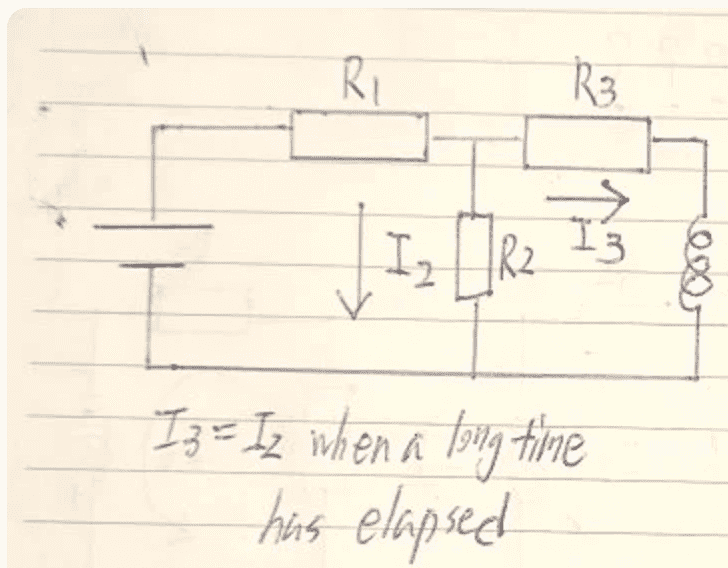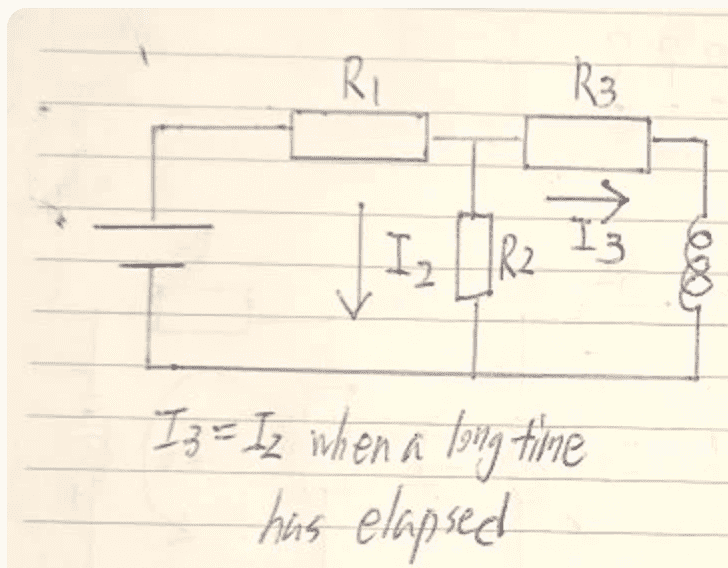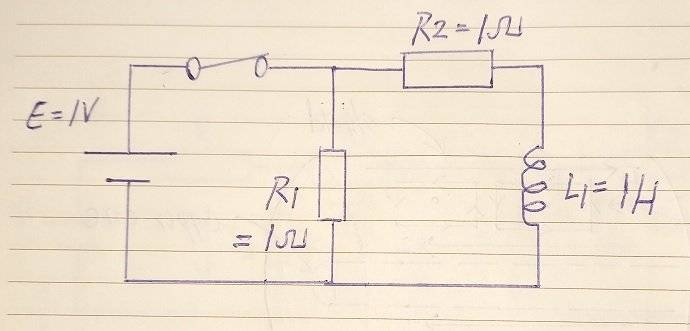# Current of RL circuit

• annamal
What is the current through the inductor right before event (c)? Does ##R_1## feature in that? Can that current change instantly to ##\frac{V}{R_2+R_3}## right after event (c)?
So is R2 parallel to R1? R1' = 1/[(1/R2) + (1/R1)], Rtotal = R1' + R3; if I(0) = V/(Rtotal) that does not give the correct current either

I find it remarkable that after an entire page of posts the OP still refuses to define the direction of his currents. Without properly taking current directions into account and with just handwaving arguments like ”currents have to be the same” without any reference to current direction, we will not progress.

Another fundamental fact that the OP ignores is that current through an inductor changes continuously. This is the same type of issue that was treated in another of OP’s recent threads.

It is unclear why the OP believes that the initial current should be ##V/(R_2+R_3)##. Yes, the closed loop only contains R2 and R3 after the switch opens, but it does not contain the EMF.

•SammyS, jim mcnamara and alan123hk
So is R2 parallel to R1? R1' = 1/[(1/R2) + (1/R1)], Rtotal = R1' + R3; if I(0) = V/(Rtotal) that does not give the correct current either
No. You need to review how inductors behave in the short and long term limits. In the short term an inductor keeps its current (including the current direction!) and in the long term it acts as a perfect conductor (as long as we are only considering direct currents).

Allegedly you have already answered (b). What is the current through the inductor in (b) and why? This current will not change directly after opening the switch. What does this tell you about ##I_2## right after opening the switch?

•SammyS
So is R2 parallel to R1? R1' = 1/[(1/R2) + (1/R1)], Rtotal = R1' + R3; if I(0) = V/(Rtotal) that does not give the correct current either
Seems our brains are in different "phase" here . Please calculate ##R_{total}## with the circuit configuration as it is at the end of (b), that is before the switch reopens and when inductor acts as a short circuit (or as a perfect conductor if you prefer that).

So is R2 parallel to R1? R1' = 1/[(1/R2) + (1/R1)], Rtotal = R1' + R3; if I(0) = V/(Rtotal) that does not give the correct current either

You seem to be trying to apply Thevenin's theorem, but you don't know how to calculate Thevenin's equivalent voltage.

Anyway, I believe you should agree that the current should not change anymore after the circuit has had enough time to stabilize, at which point the inductor can be considered a perfect conductor, you can do the calculations with a very simple circuit, that is a resistor (R1) is in series with two parallel resistors (R2 and R3), so you should be able to easily find the desired inductor initial current.

This reminds me of a question you would get in an applied differential equations course. All you really have to do is make a reasonable assumption about I3 such that I1 = I2 + I3.

Suppose the switch is open and the circuit has settled down. The inductor is then a dead short so R2 and R3 are effectively connected in parallel and there is no current or voltages across the resistors ie I1, I2, I3 = 0. If the switch is closed again then after it has settled down the inductor is a dead short and currents are easily calculated.

When the switch is instantaneously open or closed the current in the inductor changes creating a voltage so the currents are more difficult to calculate. If the switch is closed V = I2*R2 = I3*R3 + L dI3/dt where V is the voltage across R2. When the switch is closed it is a step function F(t) of zero before t=0 and 50V after t=0. F(t) = I1*R1 + I2*R2 and F(t) = I1*R1 + I3*R3 + L dI3/dt. This second equation is a first-order differential equation that can be solved in a number of ways eg Laplace Transforms.

You express everything in terms of I2 and I3, take Laplace transforms and solve the resulting simultaneous equations. When reopened F(t) = 50v at before t=0 and zero after t=0. In the tradition of our forum where we believe in guiding posters rather than doing all the work that should be enough for the OP to solve the problem.

Thanks
Bill

Last edited:
When the switch is instantaneously open or closed the current in the inductor changes creating a voltage so the currents are more difficult to calculate. If the switch is closed V = I2*R2 = I3*R3 + L dI3/dt where V is the voltage across R2. When the switch is closed it is a step function F(t) of zero before t=0 and 50V after t=0. F(t) = I1*R1 + I2*R2 and F(t) = I1*R1 + I3*R3 + L dI3/dt. This second equation is a first-order differential equation that can be solved in a number of ways eg Laplace Transforms.
There is no need to solve differential equations to answer the questions being asked here. All you need to know is that on large times, the inductor acts like a short circuit and on small times the current through it remains the same (ie, it needs to change continuously).

•bob012345, bhobba and DaveE
You express everything in terms of I2 and I3, take Laplace transforms and solve the resulting simultaneous equations. When reopened F(t) = 50v at before t=0 and zero after t=0. In the tradition of our forum where we believe in guiding posters rather than doing all the work that should be enough for the OP to solve the problem.
Yes, but guiding them to use advanced techniques that they are unlikely to understand in an unnecessary effort to solve everything about the circuit response may not be very helpful.

•bhobba and Steve4Physics
Yes, but guiding them to use advanced techniques that they are unlikely to understand in an unnecessary effort to solve everything about the circuit response may not be very helpful.
100% agree. The solution to the original question is (using the right approach) almost trivial and requires only basic arithmetic. But it needs an intuition about how inductors behave (Post #23).

I would add, also at t= ∞.

•DaveE
Differential equations are used when we need to find the transient response of an LCR circuit.

I find it remarkable that after an entire page of posts the OP still refuses to define the direction of his currents. Without properly taking current directions into account and with just handwaving arguments like ”currents have to be the same” without any reference to current direction, we will not progress.
Sorry if I didn't answer your questions clearly. The directions of I2 and I3 are below. I3 = I2 when the switch is openAnother fundamental fact that the OP ignores is that current through an inductor changes continuously. This is the same type of issue that was treated in another of OP’s recent threads.
Yes, I know that. That's why in my first post I have ##\frac{LdI_2}{dt} + I_2 R_3 + I_2 R_2 = 0## where that is kirkoff's loop laws for the I2 circuit with the inductor, solving for I2, we have ##I_2 = \frac{V}{R_3 + R_2}e^{-t/\tau}## where I2 is the current going through the inductor
It is unclear why the OP believes that the initial current should be ##V/(R_2+R_3)##. Yes, the closed loop only contains R2 and R3 after the switch opens, but it does not contain the EMF.
What should the initial current be then?

Last edited:
Sorry if I didn't answer your questions clearly. The directions of I2 and I3 are below. I3 = I2 when the switch is openDo you understand the concept of polarity? |I2| = |I3| isn't the same as I2 = I3.
In your diagram if I2 = I3 then (by KCL) the current through R1 must be I2 + I3, which will be hard to achieve if the switch is open.
It looks to me, from your diagram that I2 + I3 = 0 thus I2 = -I3 when the switch is open.

•bob012345
Do you understand the concept of polarity? |I2| = |I3| isn't the same as I2 = I3.
In your diagram if I2 = I3 then (by KCL) the current through R1 must be I2 + I3, which will be hard to achieve if the switch is open.
It looks to me, from your diagram that I2 + I3 = 0 thus I2 = -I3 when the switch is open.
Yes I2 = -I3...wondering if you could help me solve the problem from here...

Just wondering is the answer key for (c) 2.54A?

Yes, I know that. That's why in my first post I have LdI2dt+I2R3+I2R2=0 where that is kirkoff's loop laws for the I2 circuit with the inductor, solving for I2, we have I2=VR3+R2e−t/τ where I2 is the current going through the inductor
You are completely missing the point.

First of all, getting the correct differential equation is not sufficient to accurately describe the system. You also need to find the correct initial conditions, which is where you are failing. Second, (c) is a question about the initial condition so the actual differential equation is irrelevant to solving (c).

What should the initial current be then?
You tell me: How did you arrive at that initial condition? What is the logical steps you took to deduce it? You have been here long enough to know that we are not in the habit of just telling you the answer.
Yes I2 = -I3...wondering if you could help me solve the problem from here...
People have been doing nothing else than trying to help you for almost 50 posts. Adding a dismissive attitude on top of refusing to define your variables for an entire page is not going to increase people’s willingness to help you.

•Delta2
Yes I2 = -I3...wondering if you could help me solve the problem from here...
I thought we were. If you are asking us to solve it for you, then probably not. The point isn't to get the answer, it's to learn how to get the answer the next time. What is it that you don't understand about your own work on this problem?

•Orodruin
Let's back up a bit and deal with some basics first. Do you know 1st year calculus? Is there anything about these (equivalent) inductor equations that you find confusing?

$$v(t) = L⋅ \frac{di(t)}{dt} \Leftrightarrow i(t) = \frac{1}{L} \int_0^t v(\tau) \, d\tau + i(0)$$

Maybe try to simplify the problem as shown below. Reopen the switch after the circuit has settled down, is it possible that the initial current of the inductor is ##~\frac{E}{R1+R_2}=\frac{1}{1+1}=0.5A ## ?